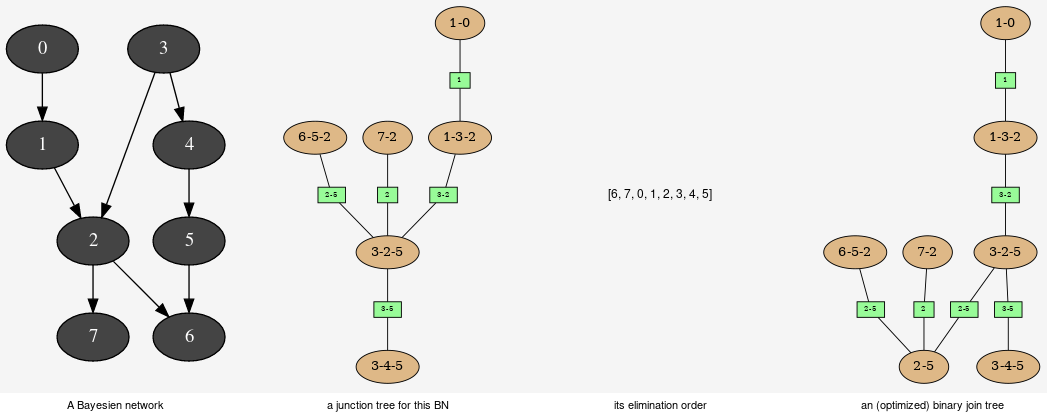# Explanation and analysis¶This tools aimed to provide some different views on the Bayesian network in order to explore its qualitative and/or quantitave behaviours.

class pyAgrum.JunctionTreeGenerator

JunctionTreeGenerator is use to generate junction tree or binary junction tree from bayesian networks.

JunctionTreeGenerator() -> JunctionTreeGenerator
default constructor
binaryJoinTree(JunctionTreeGenerator self, UndiGraph g, PyObject * partial_order=None)

binaryJoinTree(JunctionTreeGenerator self, DAG dag, PyObject * partial_order=None) -> CliqueGraph binaryJoinTree(JunctionTreeGenerator self, BayesNet bn, PyObject * partial_order=None) -> CliqueGraph

Computes the binary joint tree for its parameters. If the first parameter is a graph, the heurisitcs assume that all the node have the same domain size (2). If given, the heuristic takes into account the partial order for its elimination order.

Parameters: g (pyAgrum.UndiGraph) – a undirected graph dag (pyAgrum.DAG) – a dag bn (pyAgrum.BayesNet) – a BayesianNetwork partial_order (List[List[int]]) – a partial order among the nodeIDs the current binary joint tree pyAgrum.CliqueGraph
eliminationOrder(JunctionTreeGenerator self, UndiGraph g, PyObject * partial_order=None)

eliminationOrder(JunctionTreeGenerator self, DAG dag, PyObject * partial_order=None) -> PyObject eliminationOrder(JunctionTreeGenerator self, BayesNet bn, PyObject * partial_order=None) -> PyObject

Computes the elimination for its parameters. If the first parameter is a graph, the heurisitcs assume that all the node have the same domain size (2). If given, the heuristic takes into account the partial order for its elimination order.

Parameters: g (pyAgrum.UndiGraph) – a undirected graph dag (pyAgrum.DAG) – a dag bn (pyAgrum.BayesNet) – a BayesianNetwork partial_order (List[List[int]]) – a partial order among the nodeIDs the current elimination order. pyAgrum.CliqueGraph
junctionTree(JunctionTreeGenerator self, UndiGraph g, PyObject * partial_order=None)

junctionTree(JunctionTreeGenerator self, DAG dag, PyObject * partial_order=None) -> CliqueGraph junctionTree(JunctionTreeGenerator self, BayesNet bn, PyObject * partial_order=None) -> CliqueGraph

Computes the junction tree for its parameters. If the first parameter is a graph, the heurisitcs assume that all the node have the same domain size (2). If given, the heuristic takes into account the partial order for its elimination order.

Parameters: g (pyAgrum.UndiGraph) – a undirected graph dag (pyAgrum.DAG) – a dag bn (pyAgrum.BayesNet) – a BayesianNetwork partial_order (List[List[int]]) – a partial order among the nodeIDs the current junction tree. pyAgrum.CliqueGraph
class pyAgrum.EssentialGraph(*args)

Proxy of C++ pyAgrum.EssentialGraph class.

arcs(EssentialGraph self)
Returns: The lisf of arcs in the EssentialGraph list
children(EssentialGraph self, int id)
Parameters: id (int) – the id of the parent the set of all the children Set
connectedComponents()

connected components from a graph/BN

Compute the connected components of a pyAgrum’s graph or Bayesian Network (more generally an object that has nodes, children/parents or neighbours methods)

The firstly visited node for each component is called a ‘root’ and is used as a key for the component. This root has been arbitrarily chosen during the algorithm.

Parameters: graph (pyAgrum's graph) – A graph, a Bayesian network, more generally an object that has nodes, children/parents or neighbours methods in which the search will take place dict of connected components (as set of nodeIds (int)) with a nodeId (root) of each component as key. dict(int,Set[int])
edges(EssentialGraph self)
Returns: the list of the edges List
ids()

Deprecated method in pyAgrum>0.12.0. See nodes instead.

mixedGraph(EssentialGraph self)
Returns: the mixed graph pyAgrum.MixedGraph
neighbours(EssentialGraph self, int id)
Parameters: id (int) – the id of the checked node The set of edges adjacent to the given node Set
nodes(EssentialGraph self)
parents(EssentialGraph self, int id)
Parameters: id – The id of the child node the set of the parents ids. Set
size(EssentialGraph self)
Returns: the number of nodes in the graph int
sizeArcs(EssentialGraph self)
Returns: the number of arcs in the graph int
sizeEdges(EssentialGraph self)
Returns: the number of edges in the graph int
sizeNodes(EssentialGraph self)
Returns: the number of nodes in the graph int
skeleton(EssentialGraph self)
toDot(EssentialGraph self)
Returns: a friendly display of the graph in DOT format str
class pyAgrum.MarkovBlanket(*args)

Proxy of C++ pyAgrum.MarkovBlanket class.

arcs(MarkovBlanket self)
Returns: the list of the arcs List
children(MarkovBlanket self, int id)
Parameters: id (int) – the id of the parent the set of all the children Set
connectedComponents()

connected components from a graph/BN

Compute the connected components of a pyAgrum’s graph or Bayesian Network (more generally an object that has nodes, children/parents or neighbours methods)

The firstly visited node for each component is called a ‘root’ and is used as a key for the component. This root has been arbitrarily chosen during the algorithm.

Parameters: graph (pyAgrum's graph) – A graph, a Bayesian network, more generally an object that has nodes, children/parents or neighbours methods in which the search will take place dict of connected components (as set of nodeIds (int)) with a nodeId (root) of each component as key. dict(int,Set[int])
dag(MarkovBlanket self)
Returns: a copy of the DAG pyAgrum.DAG
hasSameStructure(MarkovBlanket self, DAGmodel other)
Parameters: pyAgrum.DAGmodel – a direct acyclic model True if all the named node are the same and all the named arcs are the same bool
nodes(MarkovBlanket self)
Returns: the set of ids set
parents(MarkovBlanket self, int id)
Parameters: id – The id of the child node the set of the parents ids. Set
size(MarkovBlanket self)
Returns: the number of nodes in the graph int
sizeArcs(MarkovBlanket self)
Returns: the number of arcs in the graph int
sizeNodes(MarkovBlanket self)
Returns: the number of nodes in the graph int
toDot(MarkovBlanket self)
Returns: a friendly display of the graph in DOT format str